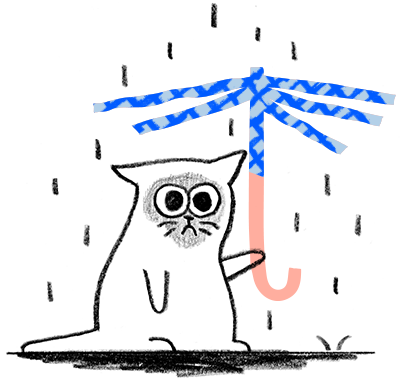cancel
Showing results for
Did you mean:
English
Announcements
Update: Find information on Dropbox support during COVID-19 here
Close
cancel
Showing results for
Did you mean:
HighlightedNew member | Level 1

I have an error with a file upload of ~452 MB this is the exception:

the code is picked from here

`('64cb960257abb6a107b327cfefbad8ab', 502, u'\n\nDropbox - 5xx\n\n\n\n\n\n\n\n\nError (5xx)Something went wrong. Don\'t worry, your files are still safe and the Dropboxers have been notified. Check out our Help Center and forums for help, or head back to home.\n\n\n\n\nmessage = {"ru": "\&amp;amp;amp;lt;h1\&amp;amp;amp;gt;\О\ш\и\б\к\а (5xx)\&amp;amp;amp;lt;/h1\&amp;amp;amp;gt;\П\р\о\и\з\о\ш\л\а \н\е\и\з\в\е\с\т\н\а\я \о\ш\и\б\к\а. \Н\е \б\е\с\п\о\к\о\й\т\е\с\ь, \в\а\ш\и \ф\а\й\л\ы \н\е \п\о\с\т\р\а\д\а\л\и, \а \с\о\т\р\у\д\н\и\к\и Dropbox \п\о\л\у\ч\и\л\и \у\в\е\д\о\м\л\е\н\и\е. \П\е\р\е\й\д\и\т\е \в \&amp;amp;amp;lt;a href=\\"https://www.dropbox.com/help\\"\&amp;amp;amp;gt;\с\п\р\а\в\о\ч\н\ы\й \ц\е\н\т\р\&amp;amp;amp;lt;/a\&amp;amp;amp;gt; \и\л\и \н\а \&amp;amp;amp;lt;a href=\\"https://forums.dropbox.com\\"\&amp;amp;amp;gt;\ф\о\р\у\м\ы\&amp;amp;amp;lt;/a\&amp;amp;amp;gt; \д\л\я \п\о\л\у\ч\е\н\и\я \п\о\м\о\щ\и \и\л\и \в\е\р\н\и\т\е\с\ь \н\а \&amp;amp;amp;lt;a href=\\"https://www.dropbox.com/home\\"\&amp;amp;amp;gt;\д\о\м\а\ш\н\ю\ю \с\т\р\а\н\и\ц\у\&amp;amp;amp;lt;/a\&amp;amp;amp;gt;.", "fr": "\&amp;amp;amp;lt;h1\&amp;amp;amp;gt;Erreur (5xx)\&amp;amp;amp;lt;/h1\&amp;amp;amp;gt;Un probl\ème est survenu. Ne vous inqui\étez pas, vos fichiers sont toujours en s\écurit\é et les Dropboxeurs ont \ét\é avertis. Consultez notre \&amp;amp;amp;lt;a href=\\"https://www.dropbox.com/help\\"\&amp;amp;amp;gt;centre d\'assistance\&amp;amp;amp;lt;/a\&amp;amp;amp;gt; et nos \&amp;amp;amp;lt;a href=\\"https://forums.dropbox.com\\"\&amp;amp;amp;gt;forums\&amp;amp;amp;lt;/a\&amp;amp;amp;gt; pour obtenir de l\'aide, ou retournez sur la page d\'\&amp;amp;amp;lt;a href=\\"https://www.dropbox.com/home\\"\&amp;amp;amp;gt;accueil\&amp;amp;amp;lt;/a\&amp;amp;amp;gt;.", "nb_NO": "\&amp;amp;amp;lt;h1\&amp;amp;amp;gt;Feilmelding (5xx)\&amp;amp;amp;lt;/h1\&amp;amp;amp;gt;Noe gikk galt. Ikke bekymre deg, filene dine er trygge og Dropbox er varslet. Sjekk ut v\årt \&amp;amp;amp;lt;a href=\\"https://www.dropbox.com/help\\"\&amp;amp;amp;gt;hjelpesenter\&amp;amp;amp;lt;/a\&amp;amp;amp;gt; og \&amp;amp;amp;lt;a href=\\"https://forums.dropbox.com\\"\&amp;amp;amp;gt;fora\&amp;amp;amp;lt;/a\&amp;amp;amp;gt; for hjelp, eller g\å tilbake til \&amp;amp;amp;lt;a href=\\"https://www.dropbox.com/home\\"\&amp;amp;amp;gt;hjemmesiden\&amp;amp;amp;lt;/a\&amp;amp;amp;gt;.", "zh_TW": "\&amp;amp;amp;lt;h1\&amp;amp;amp;gt;\錯\誤 (5xx)\&amp;amp;amp;lt;/h1\&amp;amp;amp;gt;\發\生\錯\誤\。\別\擔\心\，\您\的\檔\案\安\全\無\虞\，\已\通\知 Dropbox \員\工\。\請\到\我\們\的\&amp;amp;amp;lt;a href=\\"https://www.dropbox.com/help\\"\&amp;amp;amp;gt;\說\明\中\心\&amp;amp;amp;lt;/a\&amp;amp;amp;gt;\和\&amp;amp;amp;lt;a href=\\"https://forums.dropbox.com\\"\&amp;amp;amp;gt;\討\論\區\&amp;amp;amp;lt;/a\&amp;amp;amp;gt;\取\得\協\助\，\或\是\回\到\&amp;amp;amp;lt;a href=\\"https://www.dropbox.com/home\\"\&amp;amp;amp;gt;\首\頁\&amp;amp;amp;lt;/a\&amp;amp;amp;gt;\。", "pt_BR": "\&amp;amp;amp;lt;h1\&amp;amp;amp;gt;Erro ( 5xx ).\&amp;amp;amp;lt;/h1\&amp;amp;amp;gt<img id="wink" class="emoticon emoticon-wink" src="https://mxpez29397.i.lithium.com/html/images/emoticons/1f609.png" alt="" title="" />eu algo errado, mas n\ão se preocupe. Os seus arquivos permanecem em seguran\ça, e a equipe do Dropbox j\á foi notificada. Confira nossa \&amp;amp;amp;lt;a href=\\"https://www.dropbox.com/help\\"\&amp;amp;amp;gt;Central de ajuda\&amp;amp;amp;lt;/a\&amp;amp;amp;gt; e \&amp;amp;amp;lt;a href=\\"https://forums.dropbox.com\\"\&amp;amp;amp;gt;nossos f\óruns\&amp;amp;amp;lt;/a\&amp;amp;amp;gt; para obter ajuda ou volte para a \&amp;amp;amp;lt;a href=\\"https://www.dropbox.com/home\\"\&amp;amp;amp;gt;p\ágina inicial\&amp;amp;amp;lt;/a\&amp;amp;amp;gt;.", "zh_CN": "\&amp;amp;amp;lt;h1\&amp;amp;amp;gt;\错\误 (5xx)\&amp;amp;amp;lt;/h1\&amp;amp;amp;gt; \出\现\问\题\。\别\担\心\，\您\的\文\件\仍\安\全\，\而\且 Dropbox \员\工\已\收\到\通\知\。\请\查\阅\我\们\的\&amp;amp;amp;lt;a href=\\"https://www.dropbox.com/help\\"\&amp;amp;amp;gt;\帮\助\中\心\&amp;amp;amp;lt;/a\&amp;amp;amp;gt;\和 \&amp;amp;amp;lt;a href=\\"https://forums.dropbox.com\\"\&amp;amp;amp;gt;\论\坛\&amp;amp;amp;lt;/a\&amp;amp;amp;gt;\获\取\帮\助\，\或\返\回 \&amp;amp;amp;lt;a href=\\"https://www.dropbox.com/home\\"\&amp;amp;amp;gt;\主\页\&amp;amp;amp;lt;/a\&amp;amp;amp;gt;\。", "da_DK": "\&amp;amp;amp;lt;h1\&amp;amp;amp;gt;Fejl (5xx)\&amp;amp;amp;lt;/h1\&amp;amp;amp;gt; Noget gik galt. Men ingen grund til bekymring, dine filer er sikre, og Dropboxerne har f\ået besked. Tjek vores \&amp;amp;amp;lt;a href=\\"https://www.dropbox.com/help\\"\&amp;amp;amp;gt;Hj\ælpecenter\&amp;amp;amp;lt;/a\&amp;amp;amp;gt; og \&amp;amp;amp;lt;a href=\\"https://forums.dropbox.com\\"\&amp;amp;amp;gt;forummer\&amp;amp;amp;lt;/a\&amp;amp;amp;gt; for hj\ælp, eller g\å tilbage til \&amp;amp;amp;lt;a href=\\"https://www.dropbox.com/home\\"\&amp;amp;amp;gt;start\&amp;amp;amp;lt;/a\&amp;amp;amp;gt;.", "de": "\&amp;amp;amp;lt;h1\&amp;amp;amp;gt;Fehler (5xx)\&amp;amp;amp;lt;/h1\&amp;amp;amp;gt;Etwas ist schiefgelaufen. Ihre Dateien sind aber immer noch sicher und Dropbox wurde benachrichtigt. Schauen Sie sich im \&amp;amp;amp;lt;a href=\\"https://www.dropbox.com/help\\"\&amp;amp;amp;gt;Hilfecenter\&amp;amp;amp;lt;/a\&amp;amp;amp;gt; und in den \&amp;amp;amp;lt;a href=\\"https://forums.dropbox.com\\"\&amp;amp;amp;gt;Foren\&amp;amp;amp;lt;/a\&amp;amp;amp;gt; um, wenn Sie Hilfe ben\ötigen, oder kehren Sie zur\ück auf die \&amp;amp;amp;lt;a href=\\"https://www.dropbox.com/home\\"\&amp;amp;amp;gt;Startseite\&amp;amp;amp;lt;/a\&amp;amp;amp;gt;.", "ko": "\&amp;amp;amp;lt;h1\&amp;amp;amp;gt;\오\류(5xx)\&amp;amp;amp;lt;/h1\&amp;amp;amp;gt;\문\제\가 \발\생\했\습\니\다. \파\일\은 \안\전\하\게 \보\관\되\어 \있\으\니 \안\심\하\세\요. \이 \문\제\는 Dropbox \담\당\자\에\게 \전\달\되\었\으\며, \도\움\이 \필\요\한 \경\우 Dropbox\의 \&amp;amp;amp;lt;a href=\\"https://www.dropbox.com/help\\"\&amp;amp;amp;gt;\도\움\말 \센\터\&amp;amp;amp;lt;/a\&amp;amp;amp;gt; \및 \&amp;amp;amp;lt;a href=\\"https://forums.dropbox.com\\"\&amp;amp;amp;gt;\포\럼\&amp;amp;amp;lt;/a\&amp;amp;amp;gt;\을 \참\조\하\거\나 \&amp;amp;amp;lt;a href=\\"https://www.dropbox.com/home\\"\&amp;amp;amp;gt;\홈\&amp;amp;amp;lt;/a\&amp;amp;amp;gt;\으\로 \돌\아\가\세\요.", "it": "\&amp;amp;amp;lt;h1\&amp;amp;amp;gt;Errore (5xx)\&amp;amp;amp;lt;/h1\&amp;amp;amp;gt;Qualcosa \è andato storto. Non ti preoccupare i tuoi file sono comunque al sicuro e i Dropboxer sono stati notificati. Consulta il \&amp;amp;amp;lt;a href=\\"https://www.dropbox.com/help\\"\&amp;amp;amp;gt;Centro assistenza\&amp;amp;amp;lt;/a\&amp;amp;amp;gt; e \&amp;amp;amp;lt;a href=\\"https://forums.dropbox.com\\"\&amp;amp;amp;gt;i forum\&amp;amp;amp;lt;/a\&amp;amp;amp;gt; per assistenza oppure torna alla \&amp;amp;amp;lt;a href=\\"https://www.dropbox.com/home\\"\&amp;amp;amp;gt;homepage\&amp;amp;amp;lt;/a\&amp;amp;amp;gt;.", "sv_SE": "\&amp;amp;amp;lt;h1\&amp;amp;amp;gt;Fel (5xx)\&amp;amp;amp;lt;/h1\&amp;amp;amp;gt; N\ågot gick fel. Oroa dig inte. Dina filer \är fortfarande s\äkra och Dropboxare har meddelats. Kolla in v\årt \&amp;amp;amp;lt;a href=\\"https://www.dropbox.com/help\\"\&amp;amp;amp;gt;Hj\älpcenter\&amp;amp;amp;lt;/a\&amp;amp;amp;gt; och \&amp;amp;amp;lt;a href=\\"https://forums.dropbox.com\\"\&amp;amp;amp;gt;v\åra forum\&amp;amp;amp;lt;/a\&amp;amp;amp;gt; f\ör att f\å hj\älp eller g\å tillbaka till \&amp;amp;amp;lt;a href=\\"https://www.dropbox.com/home\\"\&amp;amp;amp;gt;startsidan.\&amp;amp;amp;lt;/a\&amp;amp;amp;gt;", "uk_UA": "\&amp;amp;amp;lt;h1\&amp;amp;amp;gt;\П\о\м\и\л\к\а (5xx)\&amp;amp;amp;lt;/h1\&amp;amp;amp;gt;\Щ\о\с\ь \п\і\ш\л\о \н\е \т\а\к. \Н\е \х\в\и\л\ю\й\т\е\с\я, \в\а\ш\і \ф\а\й\л\и \в \б\е\з\п\е\ц\і, \а \п\р\а\ц\і\в\н\и\к\и Dropbox \у\ж\е \в \к\у\р\с\і \п\о\д\і\й. \П\е\р\е\г\л\я\н\ь\т\е \&amp;amp;amp;lt;a href=\\"https://www.dropbox.com/help\\"\&amp;amp;amp;gt;\Ц\е\н\т\р \д\о\в\і\д\к\и\&amp;amp;amp;lt;/a\&amp;amp;amp;gt; \т\а \&amp;amp;amp;lt;a href=\\"https://forums.dropbox.com\\"\&amp;amp;amp;gt;\ф\о\р\у\м\и\&amp;amp;amp;lt;/a\&amp;amp;amp;gt; \а\б\о \п\о\в\е\р\н\і\т\ь\с\я \н\а \&amp;amp;amp;lt;a href=\\"https://www.dropbox.com/home\\"\&amp;amp;amp;gt;\г\о\л\о\в\н\у \с\т\о\р\і\н\к\у\&amp;amp;amp;lt;/a\&amp;amp;amp;gt;.", "nl_NL": "\&amp;amp;amp;lt;h1\&amp;amp;amp;gt;Fout (5xx).\&amp;amp;amp;lt;/h1\&amp;amp;amp;gt;Er is iets fout gegaan. Maak je geen zorgen, je bestanden zijn veilig en de Dropboxers zijn op de hoogte gesteld. Kijk in ons \&amp;amp;amp;lt;a href=\\"https://www.dropbox.com/help\\"\&amp;amp;amp;gt;helpcentrum\&amp;amp;amp;lt;/a\&amp;amp;amp;gt; en de \&amp;amp;amp;lt;a href=\\"https://forums.dropbox.com\\"\&amp;amp;amp;gt;forums\&amp;amp;amp;lt;/a\&amp;amp;amp;gt; voor hulp of ga terug naar de \&amp;amp;amp;lt;a href=\\"https://www.dropbox.com/home\\"\&amp;amp;amp;gt;beginpagina\&amp;amp;amp;lt;/a\&amp;amp;amp;gt;.", "es": "\&amp;amp;amp;lt;h1\&amp;amp;amp;gt;Error (5xx).\&amp;amp;amp;lt;/h1\&amp;amp;amp;gt; Ocurri\ó un problema. No te preocupes, tus archivos est\án seguros y los empleados de Dropbox recibieron un aviso. Visita el \&amp;amp;amp;lt;a href=\\"https://www.dropbox.com/help\\"\&amp;amp;amp;gt;Centro de ayuda\&amp;amp;amp;lt;/a\&amp;amp;amp;gt; y los \&amp;amp;amp;lt;a href=\\"https://forums.dropbox.com\\"\&amp;amp;amp;gt;foros\&amp;amp;amp;lt;/a\&amp;amp;amp;gt; para obtener ayuda, o bien vuelve a la \&amp;amp;amp;lt;a href=\\"https://www.dropbox.com/home\\"\&amp;amp;amp;gt;p\ágina principal\&amp;amp;amp;lt;/a\&amp;amp;amp;gt;.", "ms": "\&amp;amp;amp;lt;h1\&amp;amp;amp;gt;Ralat (5xx)\&amp;amp;amp;lt;/h1\&amp;amp;amp;gt;Ada sesuatu yang tidak kena. Jangan risau, fail anda masih selamat dan Dropboxer telah dimaklumkan. Lihat \&amp;amp;amp;lt;a href=\\"https://www.dropbox.com/help\\"\&amp;amp;amp;gt;Pusat Bantuan\&amp;amp;amp;lt;/a\&amp;amp;amp;gt; dan \&amp;amp;amp;lt;a href=\\"https://forums.dropbox.com\\"\&amp;amp;amp;gt;forum\&amp;amp;amp;lt;/a\&amp;amp;amp;gt; kami untuk bantuan, atau kembali ke \&amp;amp;amp;lt;a href=\\"https://www.dropbox.com/home\\"\&amp;amp;amp;gt;rumah\&amp;amp;amp;lt;/a\&amp;amp;amp;gt;.", "id": "\&amp;amp;amp;lt;h1\&amp;amp;amp;gt;Kesalahan(5xx)\&amp;amp;amp;lt;/h1\&amp;amp;amp;gt;Ada yang salah. Jangan cemas, file Anda tetap aman dan Dropboxer sudah diberi tahu. Kunjungi \&amp;amp;amp;lt;a href=\\"https://www.dropbox.com/help\\"\&amp;amp;amp;gt;Pusat Bantuan\&amp;amp;amp;lt;/a\&amp;amp;amp;gt; dan \&amp;amp;amp;lt;a href=\\"https://forums.dropbox.com\\"\&amp;amp;amp;gt;forum\&amp;amp;amp;lt;/a\&amp;amp;amp;gt; kami untuk mendapatkan bantuan, atau kembalilah ke \&amp;amp;amp;lt;a href=\\"https://www.dropbox.com/home\\"\&amp;amp;amp;gt;halaman awal\&amp;amp;amp;lt;/a\&amp;amp;amp;gt;.", "th_TH": "\&amp;amp;amp;lt;h1\&amp;amp;amp;gt;\ข\้\อ\ผ\ิ\ด\พ\ล\า\ด 5xx\&amp;amp;amp;lt;/h1\&amp;amp;amp;gt;\ม\ี\บ\า\ง\อ\ย\่\า\ง\ท\ี\่\ไ\ม\่\ถ\ู\ก\ต\้\อ\ง \ไ\ม\่\ต\้\อ\ง\เ\ป\็\น\ห\่\ว\ง \ไ\ฟ\ล\์\ข\อ\ง\ค\ุ\ณ\ย\ั\ง\ป\ล\อ\ด\ภ\ั\ย\แ\ล\ะ Dropboxer \ไ\ด\้\ร\ั\บ\ก\า\ร\แ\จ\้\ง\เ\ต\ื\อ\น\แ\ล\้\ว \โ\ป\ร\ด\ด\ู\ร\า\ย\ล\ะ\เ\อ\ี\ย\ด\ท\ี\่ \&amp;amp;amp;lt;a href=\\"https://www.dropbox.com/help\\"\&amp;amp;amp;gt;\ศ\ู\น\ย\์\ค\ว\า\ม\ช\่\ว\ย\เ\ห\ล\ื\อ\&amp;amp;amp;lt;/a\&amp;amp;amp;gt; \แ\ล\ะ \&amp;amp;amp;lt;a href=\\"https://forums.dropbox.com\\"\&amp;amp;amp;gt;\ฟ\อ\ร\ั\ม\&amp;amp;amp;lt;/a\&amp;amp;amp;gt; \เ\พ\ื\่\อ\ข\อ\ค\ว\า\ม\ช\่\ว\ย\เ\ห\ล\ื\อ \ห\ร\ื\อ\ก\ล\ั\บ\ไ\ป\ท\ี\่ \&amp;amp;amp;lt;a href=\\"https://www.dropbox.com/home\\"\&amp;amp;amp;gt;\ห\น\้\า\แ\ร\ก\&amp;amp;amp;lt;/a\&amp;amp;amp;gt;", "es_ES": "\&amp;amp;amp;lt;h1\&amp;amp;amp;gt;Error (5xx)\&amp;amp;amp;lt;/h1\&amp;amp;amp;gt;Se ha producido un error. No te preocupes, los archivos est\án a salvo y los Dropboxers est\án al tanto. Visita nuestro \&amp;amp;amp;lt;a href=\\"https://www.dropbox.com/help\\"\&amp;amp;amp;gt;Centro de ayuda\&amp;amp;amp;lt;/a\&amp;amp;amp;gt; y los \&amp;amp;amp;lt;a href=\\"https://forums.dropbox.com\\"\&amp;amp;amp;gt;foros\&amp;amp;amp;lt;/a\&amp;amp;amp;gt; si necesitas que te echen una mano, o bien vuelve a la \&amp;amp;amp;lt;a href=\\"https://www.dropbox.com/home\\"\&amp;amp;amp;gt;p\ágina principal\&amp;amp;amp;lt;/a\&amp;amp;amp;gt;.", "ja": "\&amp;amp;amp;lt;h1\&amp;amp;amp;gt;\エ\ラ\ー\（5xx\）\が\発\生\し\ま\し\た\。\&amp;amp;amp;lt;/h1\&amp;amp;amp;gt;\フ\ァ\イ\ル\は\安\全\で\す\の\で\ご\心\配\い\り\ま\せ\ん\。Dropboxer \に\は\既\に\通\知\さ\れ\て\い\ま\す\。\&amp;amp;amp;lt;a href=\\"https://www.dropbox.com/help\\"\&amp;amp;amp;gt;\ヘ\ル\プ\セ\ン\タ\ー\&amp;amp;amp;lt;/a\&amp;amp;amp;gt;\や\&amp;amp;amp;lt;a href=\\"https://forums.dropbox.com\\"\&amp;amp;amp;gt;\フ\ォ\ー\ラ\ム\&amp;amp;amp;lt;/a\&amp;amp;amp;gt;\を\参\照\す\る\か\、[\&amp;amp;amp;lt;a href=\\"https://www.dropbox.com/home\\"\&amp;amp;amp;gt;\ホ\ー\ム\&amp;amp;amp;lt;/a\&amp;amp;amp;gt<img id="wink" class="emoticon emoticon-wink" src="https://mxpez29397.i.lithium.com/html/images/emoticons/1f609.png" alt="" title="" /> \ペ\ー\ジ\に\戻\っ\て\く\だ\さ\い\。", "pl": "\&amp;amp;amp;lt;h1\&amp;amp;amp;gt;B\ł\ąd (5xx)\&amp;amp;amp;lt;/h1\&amp;amp;amp;gt;Wyst\ąpi\ły problemy. Nie martw si\ę, Twoje pliki s\ą nadal bezpieczne, a pracownicy us\ługi Dropbox zostali powiadomieni. Odwied\ź \&amp;amp;amp;lt;a href=\\"https://www.dropbox.com/help\\"\&amp;amp;amp;gt;Centrum pomocy\&amp;amp;amp;lt;/a\&amp;amp;amp;gt; i \&amp;amp;amp;lt;a href=\\"https://forums.dropbox.com\\"\&amp;amp;amp;gt;fora\&amp;amp;amp;lt;/a\&amp;amp;amp;gt;, aby uzyska\ć pomoc, lub przejd\ź do \&amp;amp;amp;lt;a href=\\"https://www.dropbox.com/home\\"\&amp;amp;amp;gt;strony g\ł\ównej\&amp;amp;amp;lt;/a\&amp;amp;amp;gt;."};\nfunction read_cookie (name) {\n var nameEQ = name + "=";\n var ca = document.cookie.split(\';\');\n for (var i = 0; i &amp;amp;amp;lt; ca.length; i++) {\n var c = ca[i];\n while (c.charAt(0) == \' \') {\n c = c.substring(1, c.length);\n }\n if (c.indexOf(nameEQ) === 0) {\n return c.substring(nameEQ.length, c.length);\n }\n }\n return null;\n}\nEvent.observe(document, \'dom:loaded\', function () {\n var locale = read_cookie(\'locale\');\n if (locale) {\n var msg = message[locale];\n if (msg) {\n \$(\'errorbox\').update(msg);\n }\n }\n});\n\n\n\n\n')`
2 Replies 2
Highlighted

## Re: error during upload fileNew member | Level 1

this is the code that generate the exception:

`try:    backup = open(BACKUP_DIR + '/' + backups_in_folder[-1], 'rb')    file_size = os.path.getsize(BACKUP_DIR + '/' + backups_in_folder[-1])    CHUNK_SIZE = 4 * 1024 * 1024    dbx = dropbox.Dropbox(DROPBOX_UPLOAD_ACCESSKEY)    if file_size <= CHUNK_SIZE:        dbx.files_upload(backup, '/' + backups_in_folder[-1])    else:        upload_session_start_result = dbx.files_upload_session_start(backup.read(CHUNK_SIZE))        cursor = files.UploadSessionCursor(session_id=upload_session_start_result.session_id, offset=backup.tell())        commit = files.CommitInfo(path='/' + backups_in_folder[-1])        while backup.tell() < file_size:            if (file_size - backup.tell()) <= CHUNK_SIZE:                dbx.files_upload_session_finish(backup.read(CHUNK_SIZE), cursor, commit)            else:                dbx.files_upload_session_append(backup.read(CHUNK_SIZE), cursor.session_id, cursor.offset)                cursor.offset = backup.tell()except Exception, e:    print('* ERROR:' + str(e))`
Highlighted

## Re: error during upload fileDropboxer

Hi Sergio, is this happening on every API call, or only some of them? If it's only some of them, about what percent?

## Work Smarter with Dropbox

The way we work is changing. Share and discover new ways to work smarter with Dropbox in our community.

Sound good? Let's get started.
Need more support?